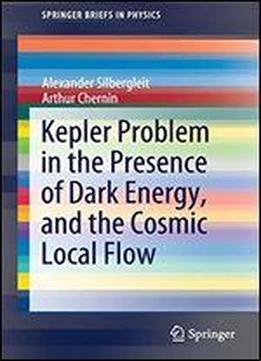# Kepler Problem In The Presence Of Dark Energy, And The Cosmic Local Flow (springerbriefs In Physics) by Alexander Silbergleit / 2020 / English / PDF

This book derives and analyzes all solutions to the Kepler problem with dark energy (DE), presenting significant results such as: (a) all radial infinite motions obey Hubbles law at large times (b) all orbital infinite motions are asymptotically radial and obey Hubbles law (c) infinite orbital motions strongly dominate the finite ones. This clearly shows the effect of repulsive DE: In the classical Kepler problem, all orbital motions are finite for negative energies and infinite in the opposite case. Another DE effect is spatial localization of bounded orbits: mostly, they are within the equilibrium sphere, where the attractive Newtonian force outbalances the repulsive force of DE. This problem is of particular current interest due to recent studies of the local flows of galaxies showing domination of DE in their dynamics the book discusses this observation in detail.

views: 212